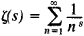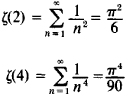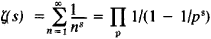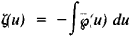# Zeta Function

(redirected from Zeta-function)

## zeta function

[′zād·ə ‚fəŋk·shən]
(mathematics)
McGraw-Hill Dictionary of Scientific & Technical Terms, 6E, Copyright © 2003 by The McGraw-Hill Companies, Inc.
The following article is from The Great Soviet Encyclopedia (1979). It might be outdated or ideologically biased.

## Zeta Function

(1) An analytic function of a complex variable s = σ + it, defined for σ > 1 by the formulaL. Euler in 1737 introduced this function in mathematical analysis for real s, and in 1859 the German mathematician G. F. B. Riemann first studied it for complex 5, and therefore it is often called the Riemann zeta function. After the works of Euler (1748, 1749), P. L. Chebyshev (1848), and Riemann the profound relation between the properties of the zeta function and the properties of prime numbers was elucidated.

Euler calculated the values of ζ(2s) for any natural s. In particularFurther, he derived the identity (the Euler identity)where the product applies to all primes p = 2, 3, 5, . . . .

The zero distribution of the zeta function is of primary importance to the theory of primes. It is known that the zeta function has zeros at the points s = -2n, where n = 1, 2, . . . (these zeros are commonly called trivial zeros) and that all other (so-called nontrivial) zeros of the zeta function lie in the strip 0 < σ < 1, which is called the critical strip. Riemann proposed that all nontrivial zeros of the zeta function lie on the straight line σ = ½ . To date this hypothesis has been neither proven nor disproven. Important results on the zero distribution of the zeta function have been obtained by a new method in analytic number theory developed by the Soviet mathematician I. M. Vinogradov.

### REFERENCES

Euler, L. Vvedenie v analiz beskonechnykh. 2nd ed., vol. 1. Moscow, 1961. (Translated from Latin.)
Wittacker, E. T., and G. Watson. Kurs sovremennogo analiza, 2nd ed., part 2. Moscow, 1963. (Translated from English.)
Titchmarsh, E. C. Dzeta-funktsiia Rimana. Moscow, 1947. (Translated from English.)
Ingham, A. E.Raspredelenie prostykh chisel Moscow-Leningrad, 1936. (Translated from English.)
Jahnke, E., and F. Emde. Tablitsy funktsii s formulami i krivymi. Moscow-Leningrad, 1948. (Translated from German.)
Prachar, K. Raspredelenie prostykh chisel. Moscow, 1967. (Translated from German.)
(2) In the theory of elliptic functions Weierstrass’ zeta function is encountered:where ζ(u) is Weierstrass’ elliptic function. This zeta function should not be confused with the Riemann zeta function.
References in periodicals archive ?
The periodic Hurwitz zeta-function [zeta](s, [alpha]; a), s = [sigma]+ it, is defined, for [sigma] > 1, by the Dirichlet series
Briggs, Some constants associated with the Riemann zeta-function, Michigan Math.
Ivic, Riemann zeta-function: theory and applications, Oversea Publishing House, 2003.
where [zeta](s) = [[SIGMA].sup.[infinity].sub.k=1][k.sup.-s] (s > 1) is the Riemann zeta-function. Concerning the computational aspects we shall derive an approximation to [gamma](a) in terms of the function [zeta](s, a), assumed to be numerically known.
 Ivic, A., 1985, The Riemann Zeta-Function, Dover, New York, NY.
where [Xi] denotes the Riemann zeta-function. This series involves the zeta-function at both positive and negative arguments.
The periodic zeta-function [zeta](s; a) is defined, for [sigma] > 1, by the Dirichlet series
Others cover Rankin-Conklin brackets on higher order modular forms, certain Eulerian integrals, the Hlawka set function, sums of integrals of powers of the zeta-function in short intervals, uniform bounds for Rankin-Selberg L-functions, mean values of zeta functions via representation theory, pair correlation of the eigenvalues of certain Laplacian elements and lower bounds for moments of L-functions with symplectic and orthogonal examples.
The Dirichlet L-function L(s, [chi]) and Hurwitz zeta-function [zeta](s, [alpha]) are defined, for [sigma] > 1, by the series
Berndt, On the Hurwitz zeta-function, Rocky Mountain J.
Really, let [zeta](s, [alpha]) denote the Hurwitz zeta-function with parameter [alpha], 0 < [alpha] [less than or equal to] 1, which, for [sigma] > 1, is given by the series

Site: Follow: Share:
Open / Close